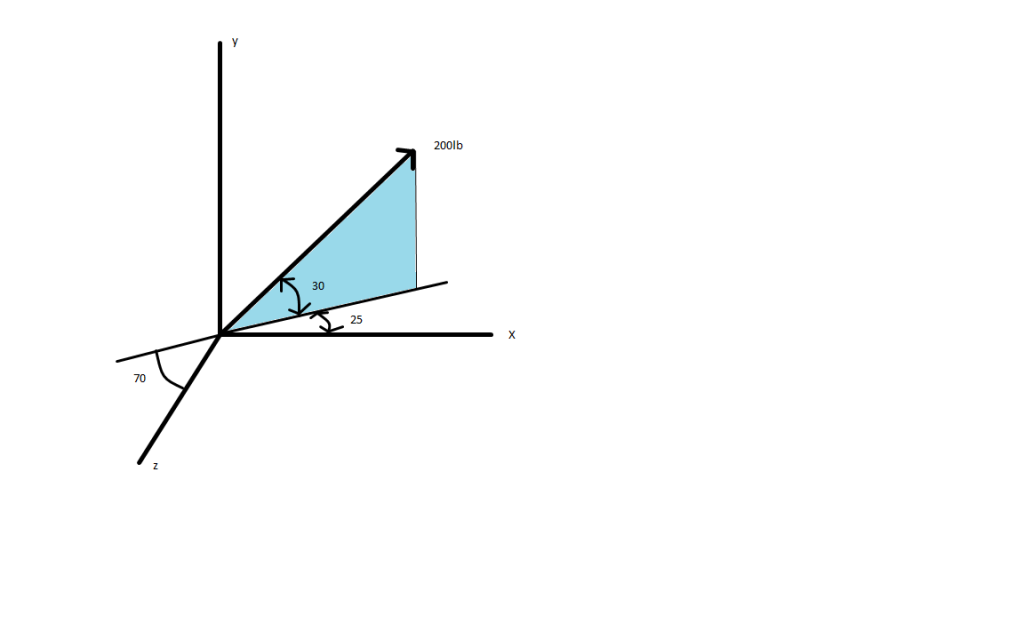User Name Remember Me? Password

 Trigonometry Trigonometry Math Forum

 December 3rd, 2009, 05:36 PM #1 Member   Joined: Mar 2009 From: San Bernardino, California Posts: 50 Thanks: 0 Direction cosine, components problem Hi everyone, it's been a while since I've posted here, but I am having a bit of trouble understanding some mathematical concepts being used in my vector mechanics class, particularly the subject of direction cosines. I'm very proficient with vectors but this particular concept is escaping me (alone with other mechanics based ones) due to the terrible nature of my book (Vector Statics for Engineers by Beer-Johnson). Here's my problem:PLEASE NOTE THE ORIENTATION OF THE AXES IN THE PICTURE ABOVE. ALL ANGLE MEASURES IN DEGREES. Now in my book, it says given the angle between a particular coordinate axis and the line of action of vector (measured from the positive axis) one can determine the components of the vector via the following formulas: $\displaystyle F_x=F\cos(\theta_x)$ $\displaystyle F_y=F\cos(\theta_y)$ $\displaystyle F_z=F\cos(\theta_z)$ In this problem, I did so and got the following: $\displaystyle F_x=200\cos(25)=$181.26 $\displaystyle F_y=200\cos(30)=$173.20 $\displaystyle F_z=200\cos(110)=$-68.40 $\displaystyle \vec {F}=<181.26, 173.20, -68.40>$ However, the answers listed by the book differ. After inspecting the solutions manual, this is what they did: $\displaystyle F_x=200\cos(30)\cos(25)=$157 $\displaystyle F_y=200\sin(30)=$1001 $\displaystyle F_z=-200\cos(30)\sin(25)=$-73.2 This I do not understand. I understand using -cos(30) in Fz and I see where I went wrong with cos(30) instead of sin(30) on Fy, but I don't see why I need to multiply by the additional trig functions in Fx and Fz. Could someone please explain this to me? Last edited by skipjack; February 28th, 2018 at 01:47 PM.December 4th, 2009, 02:21 AM #2 Senior Member   Joined: Feb 2009 From: Adelaide, Australia Posts: 1,519 Thanks: 3 Re: Direction cosine, components problem This is an exercise in using a spherical coordinate system. You are given a magnitude and two (or three) angles, and you have to deduce the Cartesian coordinates. The problem is: between what and what are the angles measured? Suppose that you start with a flat sheet of paper with an x-axis and a y-axis. If the magnitude of the vector is "r", and "a" is the angle between the vector and the x-axis, you can probably tell that the coordinates are x = r cos(a) y = r sin(a) Now suppose that you lift the pointed end of the vector of the paper, leaving the tail at the origin (0,0). If you lift it by an angle of "b" away from the paper, then z = r sin(b) but this alters the x and y values; as the vector becomes more vertical, the x and y coordinates diminish, proportionate to cos(b): x = r cos(a) cos(b) y = r sin(a) cos (b) and this gives a spherical coordinate system; not the standard one, but functional. Now, what is going on in your problem? On the basis of the solution manual, it appears that: * 30 degrees is the angle between the vector and the XZ plane (y=0), with positive angles for y>0; * If the vector is flattened onto the XZ plane (i.e. the y component is deleted), 25 degrees is the angle created, starting from the x axis and moving towards the z axis. So the angles given clearly aren't direction cosines.December 4th, 2009, 03:58 PM #3 Member   Joined: Mar 2009 From: San Bernardino, California Posts: 50 Thanks: 0 Re: Direction cosine, components problem Ok....that makes a lot of sense now. I have done spherical coordinates before and I was considering their use, but I'm not sure if that's the type of notation my professor will give credit for. You are correct in the angle measurements, I have misunderstood the idea behind a direction cosine.Tags components, cosine, direction, problemwhy sine is not used in direction cosine

Click on a term to search for related topics.
 Thread ToolsShow Printable VersionEmail this Page Display ModesLinear ModeSwitch to Hybrid ModeSwitch to Threaded ModeSimilar Threads Thread Thread Starter Forum Replies Last Post ROSJ61 Advanced Statistics 1 September 25th, 2013 06:01 PM Aimee-D Advanced Statistics 1 July 26th, 2012 04:10 AM Vesnog Trigonometry 1 February 28th, 2012 03:48 PM iMath Trigonometry 12 February 21st, 2012 12:22 AM jj007 Calculus 3 June 8th, 2010 08:57 AM

 Contact - Home - Forums - Cryptocurrency Forum - Top